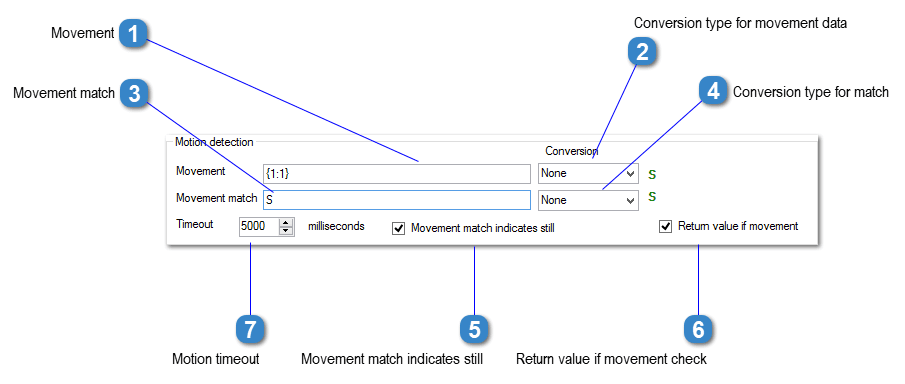# Motion DetectionThis field specifies the data that is to be compared, to determine if there is movement on the device.Use the device data buttons to assign the required data.Example:

Conversion type for movement data

Select the required conversion type. The device data is converted before the comparison is performed.

The following value conversions are available:
• 0-None – selected data from the device is assigned directly to the data field.
• 1-Value dot – selected data is a numeric string and is converted to a numeric value with ‘.’ as the decimal separator.
• 2-Value comma – selected data  is a numeric string and is converted to a numeric value with ‘,’ as the decimal separator.
• 3-IEEE754 integer – selected data is an integer in IEEE754 format (MODBUS) and is converted to a numeric value. 16,32 or 64 bit integers supported.
• 4-IEEE754 float/double dot – selected data is a number in IEEE754 format (MODBUS) and is converted to a numeric value with ‘.’ as the decimal separator. 32 bit float and 64 bit double values are supported.
• 5-IEEE754 float/double comma – selected data is a number in IEEE754 format (MODBUS) and is converted to a numeric value with ‘,’ as the decimal separator. 32 bit float and 64 bit double values are supported
• 6-Hex [0xNN] – each character in the selected string is converted to hex. E.g. ‘ABCD is converted to [0x41][0x42][0x43][0x44].
• 7-Hex 0xNNNN – the selected string is converted to hex. E.g. ‘ABCD is converted to 0x41424344.
• 8-CRC 16 – the cyclic redundancy check is calculated for the selected data.
• 9-Hex data to integer – the selected data is converted to a decimal value. e.g. ‘AA’ = 0x4141 in hex = 16705
• 10-Hex string to integer – the selected data contains the digits of a hex value. e.g. 4141 = 0x4141 = 16705

Movement match

This is the value that the movement data is compared against. The match string can be entered as a fixed value, or as part of the device data string using the device data buttons.
Fixed value can be entered as text, or in {CR}, {13} or {0x0C} formats.

Conversion type for match

Select the required conversion type. The device data is converted before the comparison is performed.

The following value conversions are available:
• 0-None – selected data from the device is assigned directly to the data field.
• 1-Value dot – selected data is a numeric string and is converted to a numeric value with ‘.’ as the decimal separator.
• 2-Value comma – selected data  is a numeric string and is converted to a numeric value with ‘,’ as the decimal separator.
• 3-IEEE754 integer – selected data is an integer in IEEE754 format (MODBUS) and is converted to a numeric value. 16,32 or 64 bit integers supported.
• 4-IEEE754 float/double dot – selected data is a number in IEEE754 format (MODBUS) and is converted to a numeric value with ‘.’ as the decimal separator. 32 bit float and 64 bit double values are supported.
• 5-IEEE754 float/double comma – selected data is a number in IEEE754 format (MODBUS) and is converted to a numeric value with ‘,’ as the decimal separator. 32 bit float and 64 bit double values are supported
• 6-Hex [0xNN] – each character in the selected string is converted to hex. E.g. ‘ABCD is converted to [0x41][0x42][0x43][0x44].
• 7-Hex 0xNNNN – the selected string is converted to hex. E.g. ‘ABCD is converted to 0x41424344.
• 8-CRC 16 – the cyclic redundancy check is calculated for the selected data.
• 9-Hex data to integer – the selected data is converted to a decimal value. e.g. ‘AA’ = 0x4141 in hex = 16705
• 10-Hex string to integer – the selected data contains the digits of a hex value. e.g. 4141 = 0x4141 = 16705

Movement match indicates still

If this box is ticked, then the scale is still if the motion data is identical to the motion match. Leave it unticked if a match indicates motion on the scale.

Return value if movement check

If at the end of the motion timeout period the scale is not still, no values will be returned to the application. Tick this box if you want the values to be returned, even if there is still motion on the scale.

Motion timeout

If there is motion on the scale, ERP-Scale will continue to read data from the device until the end of the timeout period.
Example using CRC-16 for MODBUS

In this MODBUS response the format is:
• 3 bytes of prefix.
• Float value represented in 4 bytes.
CRC-16 represented by 2 bytes The CRC-16 is calculated on the first 7 bytes of the message.

ERP-Scale can verify that the message is valid by calculating the CRC-16 value of the first 7 bytes and comparing it against the HEX representation of the last 2 bytes.
Yes No Suggest edit
Suggest Edit
Have questions? Search our knowledgebase.